#### You may also like### Calendar Capers

Choose any three by three square of dates on a calendar page...Make a set of numbers that use all the digits from 1 to 9, once and once only. Add them up. The result is divisible by 9. Add each of the digits in the new number. What is their sum? Now try some other possibilities for yourself!### Summing Consecutive Numbers

15 = 7 + 8 and 10 = 1 + 2 + 3 + 4. Can you say which numbers can be expressed as the sum of two or more consecutive integers?

# What Numbers Can We Make?

##### Age 11 to 14Challenge Level

Imagine you had four bags containing a large number of 1s, 4s, 7s and 10s.

You can choose numbers from the bags and add them to make different totals. You don't have to use numbers from every bag, and there will always be as many of each number as you need.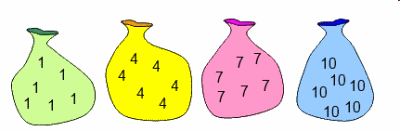Choose some sets of $3$ numbers and add them together.
Can you explain what you've noticed?

Charlie and Alison came up with some ways to represent what was happening.

Charlie's representation:

All multiples of three can be represented as: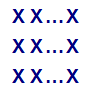The numbers in the bags can be represented as: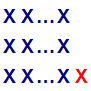Similarly, numbers which are two more than a multiple of three can be represented as: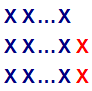When I choose three numbers, I end up with a multiple of three $+3$ which will be a multiple of three.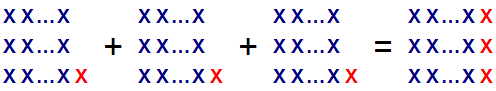Alison's representation:

Since all multiples of three can be written in the form $3n$, the numbers in the bags can be written in the form $3n+1$.

Similarly, numbers which are two more than a multiple of three can be written in the form $3n+2$.

As long as I remember I'm working with multiples of three, I could call these numbers $+0$, $+1$ and $+2$ numbers for short.

When I choose three numbers, I'm adding together three $+1$s, so I end up with a multiple of three $+3$ which will be a multiple of three.

What if you choose sets of $4$ numbers and add them together?
What if you choose sets of $5$ numbers, $6$ numbers, $7$ numbers...?
What totals do you think it would be possible to make if you choose $99$ numbers? Or 100 numbers?

Can you use Charlie's and Alison's representations to convince yourself?